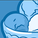Announcements
Applications are open for the 2024 Qlik Luminary Program. Apply by December 15 here.
cancel
Showing results for
Did you mean:Contributor III

## How to use if and Aggr together in expression

Hi

I have the following expression:

=sum([Amount Paid])-sum([VAT on Payment])-sum(if([VAT on Recovery]<>0,Recovery))+sum(if([VAT on Recovery]<>0,[VAT on Recovery]))

which works fine.

I am now trying to exclude certain items (i.e. Product named LB) from the calculation but I'm doing something wrong. My attempt was something like this.

=sum(Aggr(if(Product <> 'LB',(sum([Amount Paid])-sum([VAT on Payment])-sum(if([VAT on Recovery]<>0,Recovery))+sum(if([VAT on Recovery]<>0,[VAT on Recovery])))),Product))

Any assistance on how the expression should be structured will be much appreciated

1 Solution

Accepted SolutionsSpecialist

It seems to me that you could get the results you are looking by using set analysis instead of an if statement.  For the first expression try:

=sum([Amount Paid])-sum([VAT on Payment])-sum({\$<[VAT on Recovery]-={0}>} Recovery)+sum({\$<[VAT on Recovery]-={0}>} [VAT on Recovery])

And the second expression try:

=sum({\$<[Product]-={'LB'}>} [Amount Paid])-sum({\$<[Product]-={'LB'}>} [VAT on Payment])-sum({\$<[Product]-={'LB'},[VAT on Recovery]-={0}>} Recovery)+sum({\$<[Product]-={'LB'},[VAT on Recovery]-={0}>} [VAT on Recovery])

2 RepliesSpecialist

It seems to me that you could get the results you are looking by using set analysis instead of an if statement.  For the first expression try:

=sum([Amount Paid])-sum([VAT on Payment])-sum({\$<[VAT on Recovery]-={0}>} Recovery)+sum({\$<[VAT on Recovery]-={0}>} [VAT on Recovery])

And the second expression try:

=sum({\$<[Product]-={'LB'}>} [Amount Paid])-sum({\$<[Product]-={'LB'}>} [VAT on Payment])-sum({\$<[Product]-={'LB'},[VAT on Recovery]-={0}>} Recovery)+sum({\$<[Product]-={'LB'},[VAT on Recovery]-={0}>} [VAT on Recovery])Contributor III
Author

Brilliant - Much appreciatedTags
Community Browser